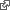#Test Counting With Method

We want to count how many times this page appears in the lineup. The question is, how to initialize the count.

The Count So Far 1 More for Here SUM The Count So Far

Now, building on this technique we imagine a cartesian space defined by floor tiles and try to dance one step at a time in a perfect circle.

10 North Tiles 10 West Tiles 0.6179 Twist Each Step

Question: What initial conditions would bring the dance around to the exact starting point in ten steps? What would make the circle round?

I try solving this experimentally in ruby.

ruby -e ' x=10; y=10; d=0.6179; 5.times { 10.times { x+=d*y; y-=d*x; puts "#{x} #{y} #{x*x+y*y}" } puts } '

I print r2 with each iteration. I'm hoping for a constant 200.

See Surprising Stability where I have explored this computation before with much smaller steps.

.

It turns out the essential magic of turtle graphics is a state machine and basic trigonometry, especially the unit circle. Given the turtle knows its own position and orientation, its next step is computed with the size of the next step multiplied by `sin` and `cos` of the orientation.

move: function move (pixels) { var p = parseFloat(pixels); this.position({ x: Math.cos(this._position.direction) * p \ + this._position.x, y: Math.sin(this._position.direction) * p \ + this._position.y }); return this; }

I took a few minutes on the bus this morning to try out Mike Bostock's newest `d3js` thing: Observable. I created a javascript table of data which includes ten steps, the computed orientation (which is a function of which step I'm on) and the `sin` and `cos` of that orientation. See observable// javascript... paste this into the browser console stepAngle = Math.PI*2/10; //10 is the number of steps data = [0, 1, 2, 3, 4, 5, 6, 7, 8, 9, 10].map( (step) => { return { cos: Math.cos(stepAngle*step), sin: Math.sin(stepAngle*step), cosSqSinSq: Math.cos(stepAngle*step)**2 + Math.sin(stepAngle*step)**2, } })

Not sure yet how to model this with the method plugin. Might need to add support for `sin` and `cos` to the markup.

.

It is exactly my resistance to employ trig that makes this a challenging problem. I'm reaching back to the early days of computer graphics where one would use feedback loops between registers to draw lines and curves.

Bresenham's line algorithm is an algorithm that determines the points of an n-dimensional raster that should be selected in order to form a close approximation to a straight line between two points. It is commonly used to draw line primitives in a bitmap image as it uses only integer addition, subtraction and bit shifting, all of which are very cheap operations in standard computer architectures. It is an incremental error algorithm. It is one of the earliest algorithms developed in the field of computer graphics. An extension to the original algorithm may be used for drawing circles. wikipediaThat last sentence, an extension for drawing circles, is what I want to implement with the Method plugin as an easter egg in the game.

So I liked your idea of just doing the trig to find the answer and then try to work back from that to get the coefficients that could be applied in each step. I just guessed from symmetry that I could use the same coefficient in x and y and for every step. This seems not to be true except possibly in very special cases.

I modified your program to compute cx and cy, the observed scale factors present in your generated circle.

stepAngle = Math.PI*2/10 s = (step) => Math.sin(stepAngle*step) c = (step) => Math.cos(stepAngle*step) data = [0, 1, 2, 3, 4, 5, 6, 7, 8, 9, 10].map( (step) => { return { x: c(step), y: s(step), cx: (c(step)-c(step-1))/s(step-1), cy: (s(step)-s(step-1))/c(step-1) } })

For this circle the cx and cy are neither equal nor uniform. But I wonder, is there a circle where this might be true.

Say you had a red roadster orbiting a planet. In a very special case that orbit would be a circle. Then with each second it advances horizontally above the surface and falls vertically under gravity. Initial conditions would have to be just right for the advance and fall to be uniform second to second.